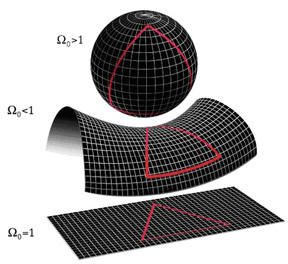A# density parameterThe density parameter (Ω) is the ratio of the actual mean density of mass-energy in the universe (ρ) to the critical densityc). A value of Ω greater than 1 implies that the universe will eventually collapse and corresponds to the case of a closed universe (i.e. one with positive curvature like that of a sphere); a value less than one suggests eternal expansion and corresponds to the case of an open universe (i.e. with negative curvature like that of the surface of saddle. The actual value remains to be determined but is thought to be close to unity (equivalent to a flat universe). See also cosmological model.